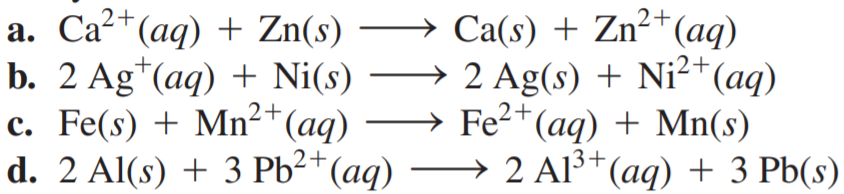×
Get Full Access to Chemistry: A Molecular Approach - 3 Edition - Chapter 18 - Problem 54e
Get Full Access to Chemistry: A Molecular Approach - 3 Edition - Chapter 18 - Problem 54e

×

# Solved: Determine whether or not each redox reactionISBN: 9780321809247 1

## Solution for problem 54E Chapter 18

Chemistry: A Molecular Approach | 3rd Edition

• Textbook Solutions
• 2901 Step-by-step solutions solved by professors and subject experts
• Get 24/7 help from StudySoup virtual teaching assistantsChemistry: A Molecular Approach | 3rd Edition

4 5 1 300 Reviews
27
5
Problem 54E

Determine whether or not each redox reaction occurs spontaneously in the forward direction.Step-by-Step Solution:
Step 1 of 3

Chemistry of Solutions Course Number: 202-NYB-05 Lecture no. 3 Date: Thursday, September 1, 2016 Professor: Nadia Schoonhoven Topics Covered: determining molar mass from Raoult's law, solution composition, deviations from Raoult's law, vapour pressure, boiling-point elevation, and freezing-point depression. Exercise: Calculate the expected vapour pressure above a solution containing 158.0 g of sucrose (table sugar, molar mass = 342.3 g) in 643.5 mL of water at 25°C. At this temperature, the density of water is 0.9971 g/mL and the vapour pressure is 23.76 torr. Given: 158.0 g of sucrose, molar mass = 342.3 g, 643.5 mL water, 25°C, density of water at 25°C is 0.9971 g/mL. Calculate the expected vapour pressure above the solution. Raoult's law equation: P solutionxsolvent° solvent We want to calculate the value of P solution We are missing the mole fraction of water (x solvent 1. Calculate the moles of sucrose in 158.0 g. 158.0 g × (1 mol. / 342.3 g) = 0.46158 mol. sucrose. 2. Calculate the moles of water in 634.5 mL. 0.9971 g/mL × 643.5 mL = 641.6339 g water 641.6993 g × 1 mol. / 18.015 g = 35.6203 mol. water. 3. Calculate the mole fraction of water. 0.46158 mol. sucrose + 35.6203 mol. water = 36.082 mol.. X = 35.6203 mol. water/ 36.082 mol. solution water = 0.98720 4. Calculate the pressure above the solution: 0 P solutionxsolvent solvent (0.9872) × (23.76 torr) = 23.46 torr. Note: keep the following plot in mind when you're asked to solve problems involving Raoult's law:

Step 2 of 3

Step 3 of 3

##### ISBN: 9780321809247

Unlock Textbook Solution